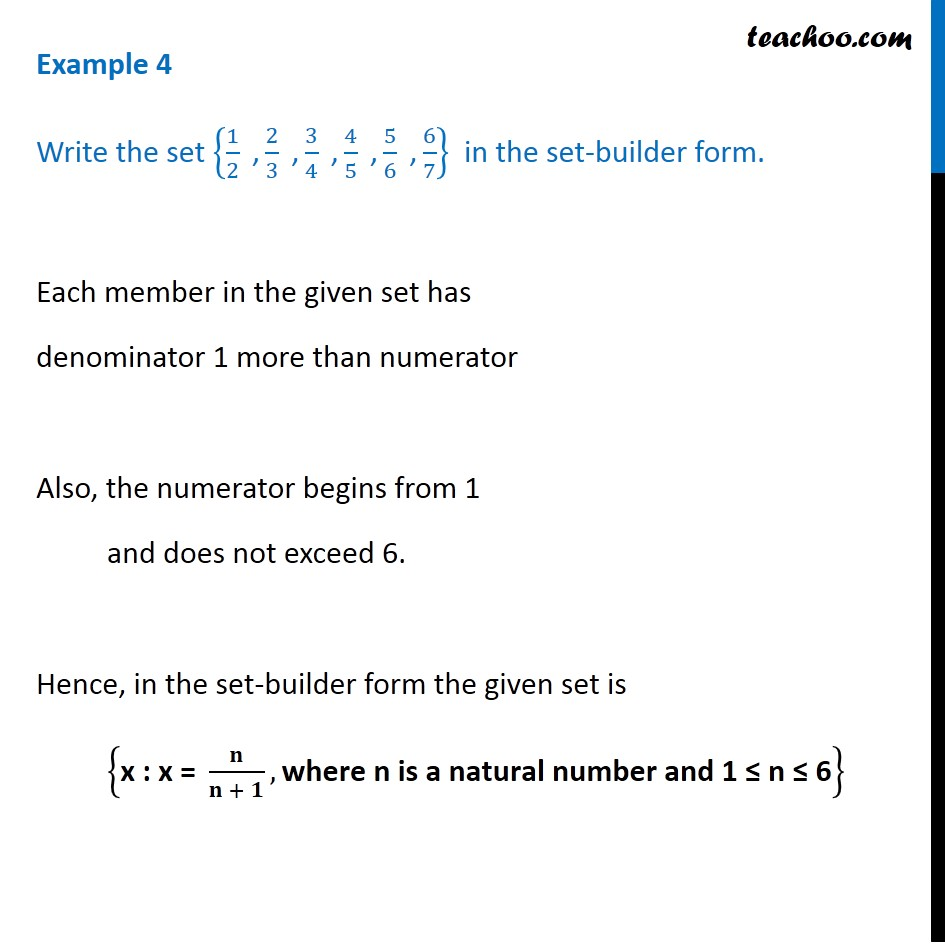1. Chapter 1 Class 11 Sets
2. Serial order wise
3. Examples

Transcript

Example 4 Write the set {1/2 " ," 2/3 " ," 3/4 " ," 4/5 " ," 5/6 " ," 6/7} in the set-builder form. Each member in the given set has denominator 1 more than numerator Also, the numerator begins from 1 and does not exceed 6. Hence, in the set-builder form the given set is {"x : x = " 𝐧/(𝐧 + 𝟏), "where n is a natural number and 1 ≤ n ≤ 6" }

Examples

Chapter 1 Class 11 Sets
Serial order wise